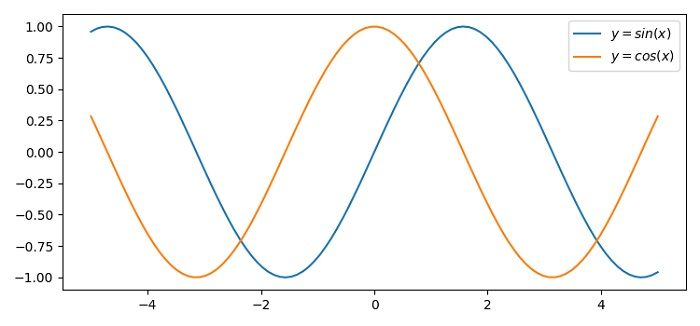# Text alignment in a Matplotlib legend

To make text alignment in a matplotlib legend, we can take the following steps −

• Set the figure size and adjust the padding between and around the subplots.

• Create x data points using numpy.

• Plot x, sin(x) and cos(x) using plot() method.

• Place legend using legend() method and initialize a method.

• Iterate the legend.get_texts() method to set the horizontal alignment.

• To display the figure, use show() method.

## Example

import numpy as np
from matplotlib import pyplot as plt

plt.rcParams["figure.figsize"] = [7.50, 3.50]
plt.rcParams["figure.autolayout"] = True

x = np.linspace(-5, 5, 100)

plt.plot(x, np.sin(x), label="$y=sin(x)$")
plt.plot(x, np.cos(x), label="$y=cos(x)$")

legend = plt.legend(loc='upper right')

for t in legend.get_texts():
t.set_ha('left')

plt.show()

## Output